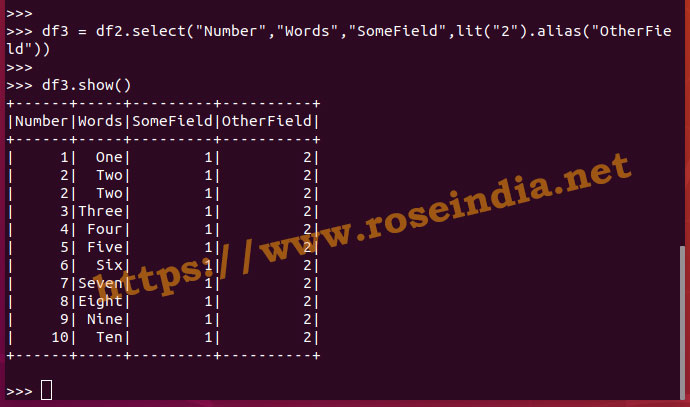Tutorials

# PySpark lit Function

How to use the PySpark lit Function in your PySpark program to add a new column to the DataFrame?

How to use the PySpark lit Function in your PySpark program to add a new column to the DataFrame?

## PySpark lit Function - pyspark.sql.functions.lit example

How to use lit function of Spark SQL to add a new column with some value to existing DataFrame?

In this example we will see the use of lit function of Spark SQL which is easy to use and most frequently used by developers to add a literal value to a DataFrame. The lit() function is from pyspark.sql.functions package of PySpark library and used to add a new column to PySpark Dataframe by assigning a static or literal value to the field.If you use this function then a new column is added to the DataFramework by assigning the static or literal value.

To use the lit function in your PySpark program you have to import in your program. Here is the code for importing the lit function in your Python program:

from pyspark.sql.functions import lit

The syntax of the functions is:

lit("value")

You can apply to DataFramewith following code:

``df.withColumn("NewColumnName", lit("Value for Column"))``

Here is the complete example program:

``````
from pyspark.sql import SparkSession
from pyspark.sql.types import ArrayType, StructField, StructType, StringType, IntegerType
from pyspark.sql.functions import col,lit

data = [(1, "One"),
(2, "Two"),
(2, "Two"),
(3, "Three"),
(4, "Four"),
(5, "Five"),
(6, "Six"),
(7, "Seven"),
(8, "Eight"),
(9, "Nine"),
(10, "Ten")]

# Create a schema for the dataframe
schema = StructType([
StructField('Number', IntegerType(), True),
StructField('Words', StringType(), True)
])

# Convert list to RDD
rdd = spark.sparkContext.parallelize(data)

# Create data frame
df = spark.createDataFrame(rdd,schema)
print(df.schema)
df.show()

df2 = df.withColumn("SomeField",lit("1"))

df2.show()

df3 = df2.select("Number","Words","SomeField",lit("2").alias("OtherField"))

df3.show()
``````

In our program following code creates a new column with literal value in our program:

``df2 = df.withColumn("SomeField",lit("1"))``

The lit() can also be used with the select statement in DataFrame. Here is one example:

``````df3 = df2.select("Number","Words","SomeField",lit("2").alias("OtherField"))
df3.show()``````

So, this way you can use the lit() function in your PySpark program.

In this tutorial we have learned to use the lit() function of PySpark for adding a new column to Spark DataFrame. You can use this function with the withColumn() and select() function of Dataframe. This is very powerful function in the hand of PySpark developers and used very frequently while processing the data.